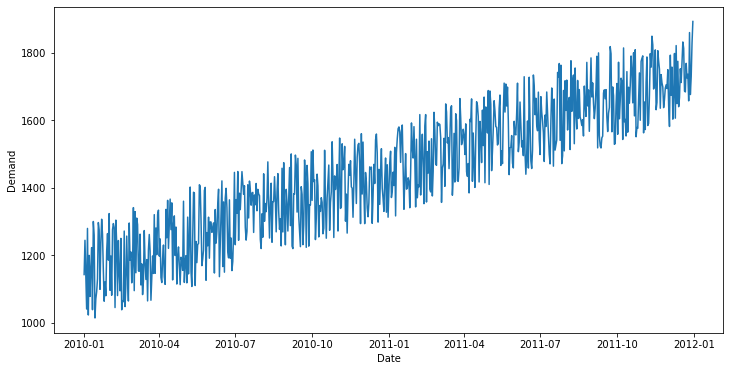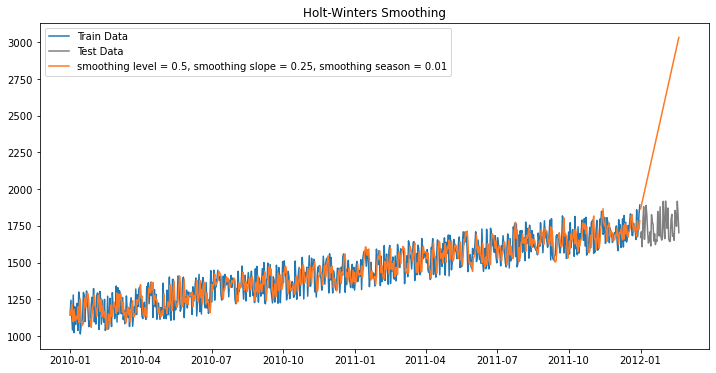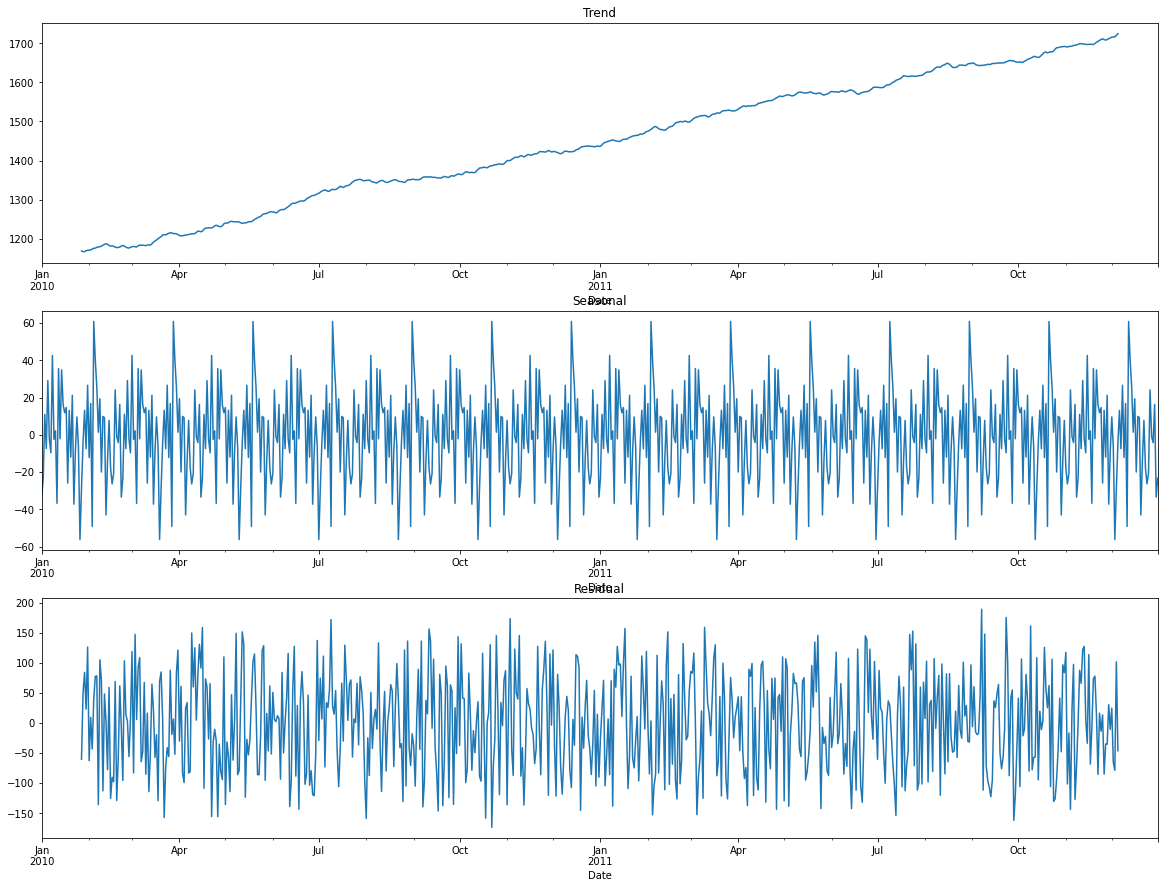# Python Forecasting Toolbox¶

This notebook presents a number of python modules built in the statsmodels package that help us automise our forecasting.

## Setting up¶

In :
!pip install pandas matplotlib numpy statsmodels

Requirement already satisfied: pandas in /opt/anaconda3/lib/python3.8/site-packages (1.1.3)
Requirement already satisfied: matplotlib in /opt/anaconda3/lib/python3.8/site-packages (3.2.2)
Requirement already satisfied: numpy in /opt/anaconda3/lib/python3.8/site-packages (1.19.2)
Requirement already satisfied: statsmodels in /opt/anaconda3/lib/python3.8/site-packages (0.11.1)
Requirement already satisfied: pytz>=2017.2 in /opt/anaconda3/lib/python3.8/site-packages (from pandas) (2020.1)
Requirement already satisfied: python-dateutil>=2.7.3 in /opt/anaconda3/lib/python3.8/site-packages (from pandas) (2.8.1)
Requirement already satisfied: kiwisolver>=1.0.1 in /opt/anaconda3/lib/python3.8/site-packages (from matplotlib) (1.2.0)
Requirement already satisfied: pyparsing!=2.0.4,!=2.1.2,!=2.1.6,>=2.0.1 in /opt/anaconda3/lib/python3.8/site-packages (from matplotlib) (2.4.7)
Requirement already satisfied: cycler>=0.10 in /opt/anaconda3/lib/python3.8/site-packages (from matplotlib) (0.10.0)
Requirement already satisfied: patsy>=0.5 in /opt/anaconda3/lib/python3.8/site-packages (from statsmodels) (0.5.1)
Requirement already satisfied: scipy>=1.0 in /opt/anaconda3/lib/python3.8/site-packages (from statsmodels) (1.5.0)
Requirement already satisfied: six>=1.5 in /opt/anaconda3/lib/python3.8/site-packages (from python-dateutil>=2.7.3->pandas) (1.15.0)

In :
import pandas as pd
import matplotlib.pyplot as plt
from statsmodels.tsa.holtwinters import SimpleExpSmoothing, Holt, ExponentialSmoothing
from statsmodels.tsa.seasonal import seasonal_decompose
from matplotlib import pyplot
import numpy as np


statsmodels.tsa.holtwinters: contains the exponential forecasting tools that we will employ in this jupyter notebook. We only import 3 different models. For more infomration on this package, we recommend you to follow these links: https://www.statsmodels.org/stable/generated/statsmodels.tsa.holtwinters.SimpleExpSmoothing.html https://www.statsmodels.org/stable/generated/statsmodels.tsa.holtwinters.Holt.html https://www.statsmodels.org/stable/generated/statsmodels.tsa.holtwinters.ExponentialSmoothing.html

This section, you can select what data is loaded by changing the data_file variable to one of three names "data1", "data2" and "data3". Each correspond to 6 years of daily demand data, with 2191 data points between 1-Jan-2010 and 31-Dec-2015.

Change start_visual and end_visual to determine what period to visualise. As reference: the start index for each 1-Jan data point are presented below: 1-Jan-2010: 0 1-Jan-2011: 365 1-Jan-2012: 730 1-Jan-2013: 1096 1-Jan-2014: 1461 1-Jan-2015: 1826

In :
data_file = 'data2' #possible values data1 / data2 / data3
start_visual = 0
end_visual = 730


### Plot data¶

Before carrying out any forecasting, we must:

1. Verify that the data is loaded properly.
2. Visually inspect the data to determine the better forecasting method.
In :
data_test = pd.read_excel(f'{data_file}.xlsx')

visualise_data = data_test.iloc[start_visual:end_visual, :]
visualise_data.set_index('Date', inplace=True)

fig, ax = plt.subplots(figsize=(12, 6))
ax.plot(visualise_data.index, visualise_data.values)
ax.set_ylabel('Demand')
ax.set_xlabel('Date')

Out:
Text(0.5, 0, 'Date')Here we utilise the pandas package to arrange and parition the data. Given that the data comes in two columns, one for the date and the other for the demand at each date, we use the set_index method to relate each demand to the corresponding date, making it easy to plot using ax.plot(visualise_data.index, visualise_data.values)

## Select Data for Forecast Training and Testing.¶

In this section, form the uploaded dataset of the previous cell, select a specific region of the data that you want to use to train the forecasting algorithms, and a section of the data that you want to test the forecast against. So choose 3 parameters: train_start, train_end, and test_end. The test data starts immediately after the training data, i.e. it will start at train_end.

In :
train_start = 0
train_end = 730
test_end = 780


## Select Forecasting Technique¶

Change the forecasting method by changing the variable forcast_method to simple exponential, holt's method or holt-winters method respectively: 'simple_exp', 'holt' and 'holt_winters' Forecasting parameters:

alpha - indicates the level coefficient (must lie between 0-1)

beta - indicates the trend coefficient (must lie between 0-1)

gamma - indicates the season coefficient (must lie between 0-1)

s_periods - indicates the number of cycles observed in the training data - only applicable to seasonal data (integer that must be larger than 1)

In :
forecast_method = 'holt_winters'

alpha = 0.5
beta = 0.25
gamma = 0.01
s_periods = 2


## Run Forecast¶

We first extract the training and testing data.

We use data_test.iloc[train_start:train_end, :] to locate the data point based on their index number.

In :
# get adequate data
train = data_test.iloc[train_start:train_end, :]
test = data_test.iloc[train_end:test_end, :]
test.set_index('Date', inplace=True)
train.set_index('Date', inplace=True)


Now we run the models. First, we create the model using the training demand:
model = SimpleExpSmoothing(np.asarray(train['Demand']))

Then, we fit our model to the demand it is associated with, using the parameters alpha beta and gamma that we specified before:
fit1 = model.fit(smoothing_level=alpha)

Finally, we use the fitted model to predict the future demand using the testing interval specified:
pred1 = fit1.forecast(test_end-train_end)

In :
# run forecasting models
if forecast_method == 'simple_exp':
model = SimpleExpSmoothing(np.asarray(train['Demand']))
# train model
fit1 = model.fit(smoothing_level=alpha)
# make prediction
pred1 = fit1.forecast(test_end-train_end)
elif forecast_method == 'holt':
model = Holt(np.asarray(train['Demand']))
fit1 = model.fit(smoothing_level=alpha, smoothing_slope=beta)
pred1 = fit1.forecast(test_end-train_end)
elif forecast_method == 'holt_winters':
fit1 = model.fit(smoothing_level=alpha, smoothing_slope=beta, smoothing_seasonal=gamma)
pred1 = fit1.forecast(test_end-train_end)


## Plot Results¶

We finally plot our forecasted and our training demand

In :
fig, ax = plt.subplots(figsize=(12, 6))
ax.plot(train.index, train.values, label='Train Data')
ax.plot(test.index, test.values, color="gray", label='Test Data')
if forecast_method == 'simple_exp':
lab = f'smoothing level = {alpha}'
elif forecast_method == 'holt':
lab = f'smoothing level = {alpha}, smoothing slope = {beta}'
elif forecast_method == 'holt_winters':
lab = f'smoothing level = {alpha}, smoothing slope = {beta}, smoothing season = {gamma}'

ax.plot(train.index, fit1.fittedvalues, color='#ff7823', label=lab)
ax.plot(test.index, pred1, color='#ff7823')

if forecast_method == 'simple_exp':
plt.title("Simple Exponential Smoothing")
elif forecast_method == 'holt':
plt.title("Holt Smoothing")
elif forecast_method == 'holt_winters':
plt.title("Holt-Winters Smoothing")

plt.legend()
plt.show()Using the seasonal_decompose method, we can decompose the time series into three different curves, ie. overall trend, seasonal patterns, and residual error.

In :
res = seasonal_decompose(visualise_data, model='additive', period=52)

fig, (ax1,ax2,ax3) = plt.subplots(3,1, figsize=(20,15))
res.trend.plot(ax=ax1, title="Trend")
res.seasonal.plot(ax=ax2, title="Seasonal")
res.resid.plot(ax=ax3, title="Residual")

Out:
<matplotlib.axes._subplots.AxesSubplot at 0x7f8eb19ae5b0>